### Distance Classification

The distance class for a point pair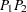is determined as follows. The directed line segmentis superimposed on the coordinate system that shows the distance or lag classes. These classes are determined by the LAGDISTANCE= option in the COMPUTE statement. Denoting the length of the line segment by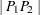and the LAGDISTANCE= value by, the lag class
is determined by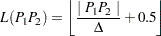where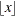denotes the largest integer.

When the directed line segmentis superimposed on the coordinate system that shows the distance classes, it is seen to fall in the first lag class; see Figure 98.21 for an illustration for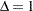.

Figure 98.21 Selected PairFalls within the First Lag ClassPairwise distances are positive. Therefore, the line segmentmight belong to one of the MAXLAG lag classes or it could be shorter than half the length of the LAGDISTANCE= value. In the last case the segment is said to belong to the lag class zero. Hence, lag class zero is smaller than lag classes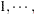MAXLAGS. The definition of lag classes in this manner means that when you specify the MAXLAGS= parameter, PROC VARIOGRAM produces a semivariogram with a total of MAXLAGS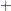1 lag classes including the zero lag class. For example, if you specify LAGDISTANCE=1 and MAXLAGS=10 and you do not specify a LAGTOLERANCE= value in the COMPUTE statement in PROC VARIOGRAM, the 11 lag classes generated by the preceding equation are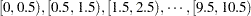The preceding lag classes description is correct under the assumption of the default lag tolerance, which is half the LAGDISTANCE= value. Using the default lag tolerance results in no gaps between the distance class intervals, as shown in Figure 98.22.

Figure 98.22 Lag Distance Axis Showing Lag ClassesOn the other hand, if you do specify a distance tolerance with the LAGTOLERANCE= option in the COMPUTE statement, a further check is performed to see whether the point pair falls within this tolerance of the nearest lag. In the preceding example, if you specify LAGDISTANCE=1 and MAXLAGS=10 (as before) and also specify LAGTOLERANCE=0.25, the intervals become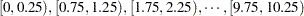You might want to avoid this specification because it results in gaps in the lag classes. For example, if a point pairfalls in an interval such as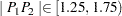then it is excluded from the semivariance calculation. The maximum LAGTOLERANCE= value allowed is half the LAGDISTANCE= value; no overlap of the distance classes is allowed.

See the section Computation of the Distribution Distance Classes for a more extensive discussion of practical aspects in the specification of the LAGDISTANCE= and MAXLAGS= options.